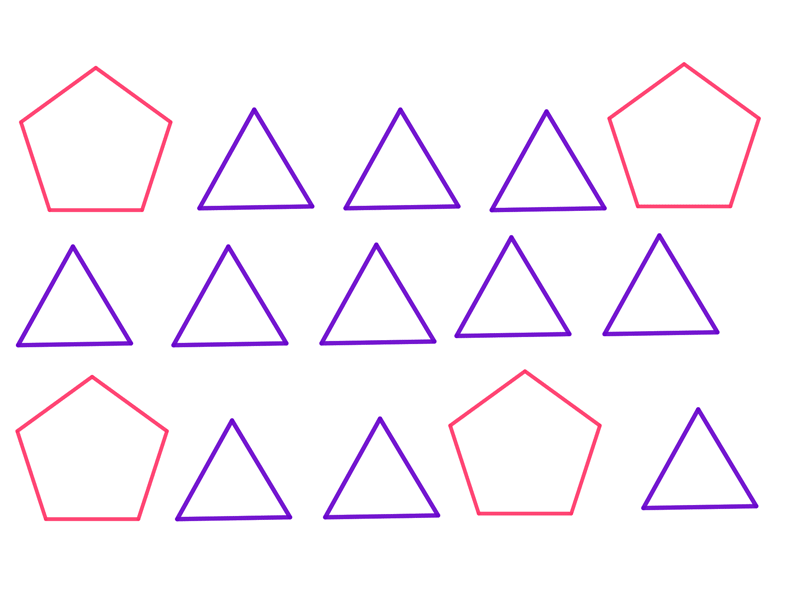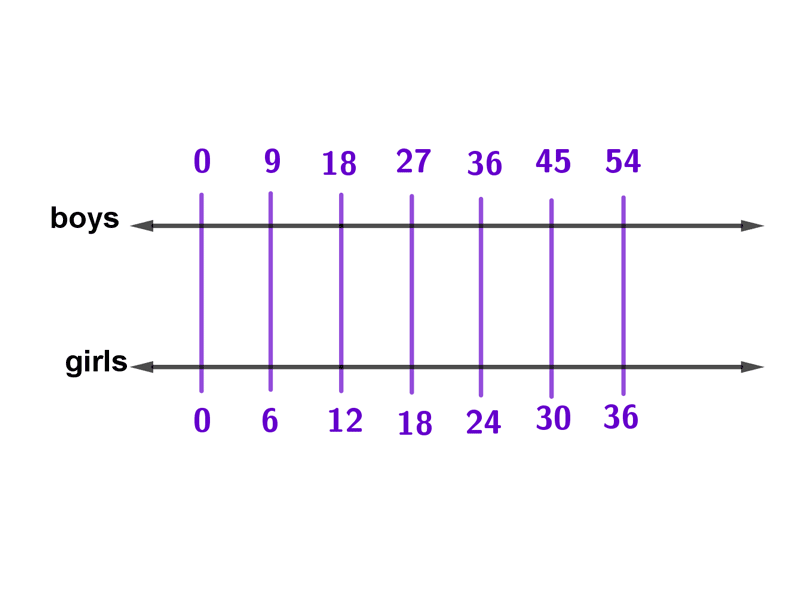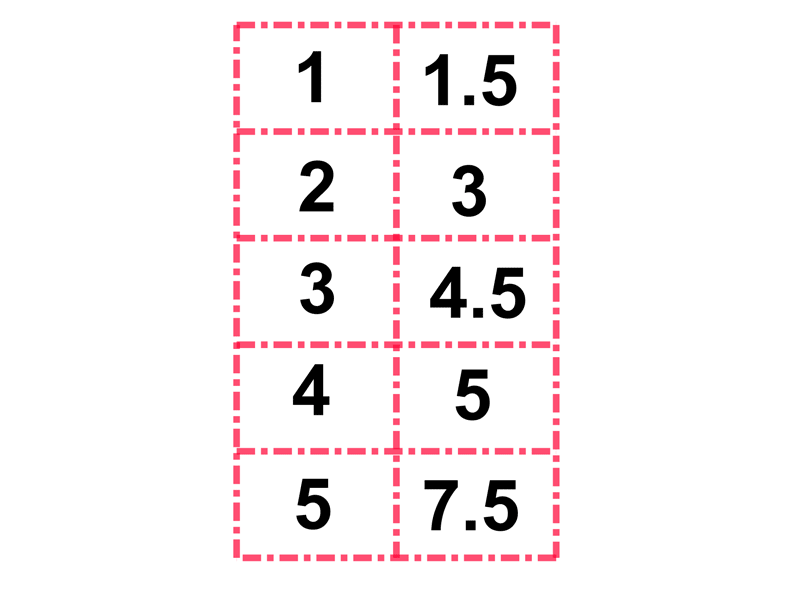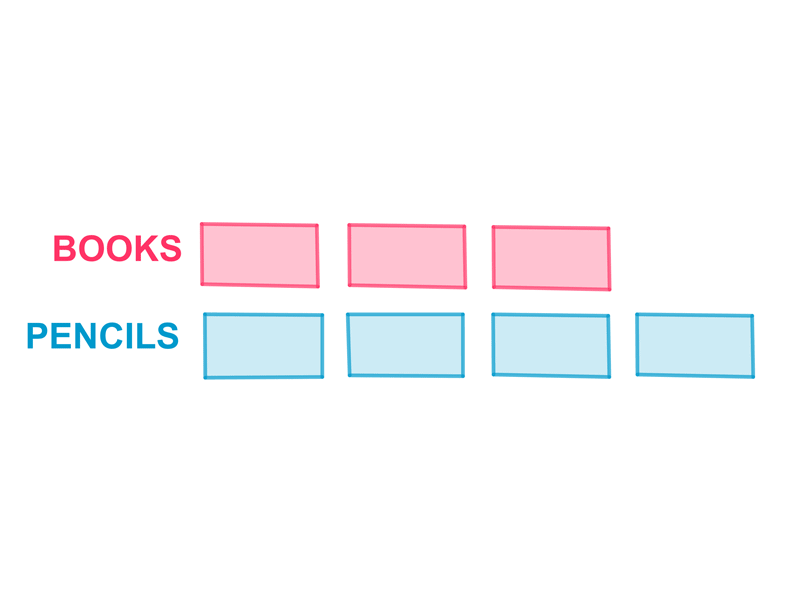Math Calculators, Lessons and Formulas

It is time to solve your math problem

mathportal.org
• Pre algebra
• Percents, Ratios and Propotions
• Ratios and rates

# Ratios and rates

ans:
syntax error
C
DEL
ANS
±
(
)
÷
×
7
8
9
4
5
6
+
1
2
3
=
0
.
auto next question
calculator
•  Question 1: 1 pts What is the ratio of pentagons to triangles?$4:15$ $15:4$ $11:4$ $4:11$
•  Question 2: 1 pts It took a pet store 9 weeks to sell 90 cats. What is the rate sold per week?
 $8$ $9$ $10$ none of these
•  Question 3: 1 pts Reduce ratio to its lowest form. $$64:72$$
 $6:8$ $8:9$ $9:8$ $9:8$
•  Question 4: 1 pts At the pet store the ratio of dogs to cats was $4:7$. For every $4$ dogs there are $7$ cats.
•  Question 5: 2 pts The ratio of red cars to blue cars in a parking lot was $6 : 3.$ If there were $40$ red cars, how many blue cars were there?
 $12$ $16$ $20$ $24$
•  Question 6: 2 pts The ratio of boys to girls at the park was 9 to 6. If there were 54 boys, how many girls were there? Use the double numberline to solve the problems.$18$ $27$ $36$ $45$
•  Question 7: 2 pts This ratio table is completed correctly.•  Question 8: 2 pts The ratio of 8 books to 20 books is
•  Question 9: 3 pts The diagram shows the ratio of books and pencils that Ann's has on her shelves. Based on the ratio, how many pencils are on the shelves if there are 96 books on the shelves?$122$ $124$ $126$ $128$
•  Question 10: 3 pts There are $'x'$ boys and$'y'$ girls in a class. The ratio of the number of boys to the total number of students in the class is:
 $\dfrac{x+y}{x}$ $\dfrac{x}{x+y}$ $\dfrac{y}{x+y}$ $\dfrac{x}{y}$
•  Question 11: 3 pts $$20g:100g=1m:500cm$$
•  Question 12: 3 pts A ratio will always be more than 1.